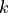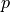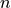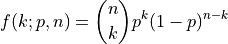# aesara.tensor.random.binomial#

aesara.tensor.random.binomial = <aesara.tensor.random.basic.BinomialRV object>[source]#

A binomial discrete random variable.

The probability mass function for `binomial` for the numberof successes in terms of the probability of successof a single trial and the numberof trials is: